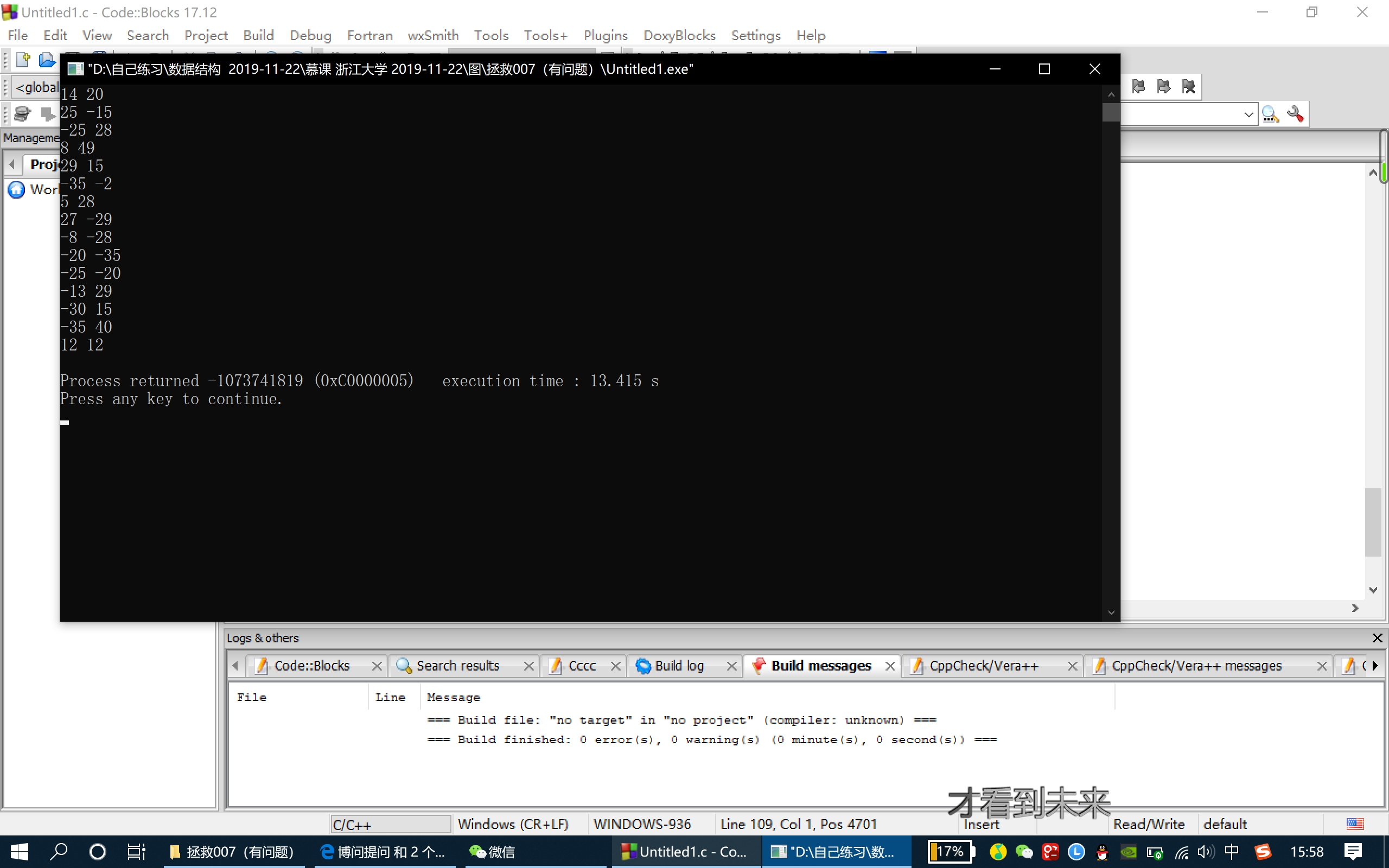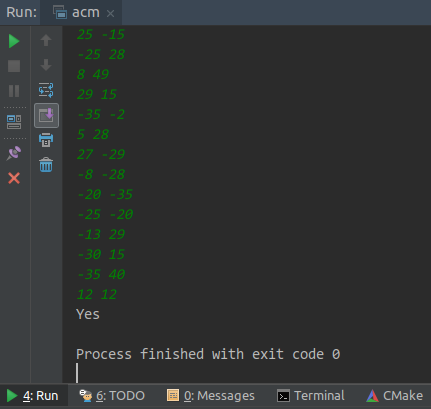•闪存
•博客
• 发言小组
• 投递新闻
• 提问博问
• 添加收藏
• 发布招聘
•文库

# for循环输入，中间如果不加输出（什么输出都行），程序错误

-2悬赏园豆：50 [已解决问题] 解决于 2020-03-01 09:59``````# include<stdio.h>
# include<math.h>
# include<stdlib.h>
# include<stdbool.h>
#define SAFE 50
struct
{
int N;              //鳄鱼数量
float D;            //能跳的最远距离
struct crocodile *C;
}Lake;      //因为只用到一个此结构体，所以直接声明并定义
struct crocodile
{
float x, y;         //横纵坐标
int v;          //是否遍历过（鳄鱼是否被踩过）， 0代表否，1代表是
};
float land = 15.0/2;

void CreateLake();
bool DFS(struct crocodile *S);
bool IsSafe(struct crocodile S);
float Distance (struct crocodile a1, struct crocodile a2);

int main()
{
int i;
scanf("%d %f", &Lake.N, &Lake.D);
CreateLake();               //建图
//for(i=0; i<=Lake.N; ++i)       //检查图创建是否正确
// printf("%f, %f, %d\n", Lake.C[i].x, Lake.C[i].y, Lake.C[i].v);
if(DFS(&(Lake.C)))  printf("Yes\n");
else  printf("No\n");
free(Lake.C);               //一定要释放
return 0;
}

void CreateLake()
{
int i;
Lake.C = malloc(Lake.N +1 * sizeof(struct crocodile));        //N+1方便运算
Lake.C.x = Lake.C.y = Lake.C.v = 0;            //C当做小岛的中心
for(i=1; i<=Lake.N; ++i){
//printf("a\n");                    //这出的问题---------------------------------------------
Lake.C[i].v = 0;
scanf("%f %f", &(Lake.C[i].x), &(Lake.C[i].y));
}
}
bool DFS(struct crocodile *S)
{
int i;
if(0==S->x && 0==S->y)          //因为小岛有直径，所以第一次跳，要加上半径，不能和其他一块进行
for(i=1; i<=Lake.N; ++i){
if(Lake.D >= (Distance(*S, Lake.C[i]) - land))
DFS(&(Lake.C[i]));
}
else{
S->v = 1;                   //遍历（跳）即标记
if(IsSafe(*S))  return true;
else{
for(i=1; i<=Lake.N; ++i){
if(!(Lake.C[i].v) && Lake.D >= Distance(*S, Lake.C[i]))
DFS(&(Lake.C[i]));
}
return false;
}

}
}
bool IsSafe(struct crocodile S)
{
if(SAFE <= fabs(S.x)+Lake.D || SAFE <= fabs(S.y)+Lake.D)
return true;
else  return false;
}
float Distance (struct crocodile a1, struct crocodile a2)
{
return sqrt(pow((a1.x-a2.x), 2) + pow((a1.y-a2.y), 2));
}``````0

haoba

り罐罐。 | 初学一级 |园豆：46 | 2020-02-22 16:14

0

Adming | 园豆：13 (初学一级) | 2019-12-09 14:13

@り罐罐。: 问题出在scanf函数上，百度一个 scanf for 就知道了。 加 printf 可以运行，相当于强制线程切换了。

@り罐罐。: 建议不要用一个scanf接收多个输入，在循环中记得用fflush(stdin);或getchar()来吸收回车

0KimBing_Ng | 园豆：352 (菜鸟二级) | 2020-02-23 08:57

您需要登录以后才能回答，未注册用户请先注册